# DAV Class 5 Maths Chapter 5 Worksheet 3 Solutions

The DAV Class 5 Maths Book Solutions Pdf and DAV Class 5 Maths Chapter 5 Worksheet 3 Solutions of Decimals offer comprehensive answers to textbook questions.

## DAV Class 5 Maths Ch 5 Worksheet 3 Solutions

Question 1.
Write in decimal form. The first one is done for you.
(a)Solution:(b)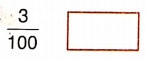Solution: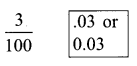(c)Solution: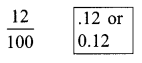(d)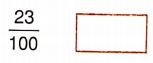Solution:(e)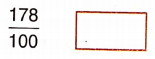Solution: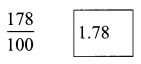(f)Solution:(g)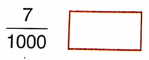Solution: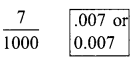(h)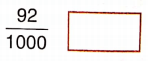Solution: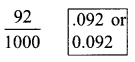(i)Solution:Question 2.
Write as a fraction. The first one is done for you.
(a)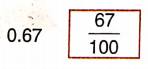Solution: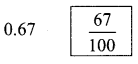(b)Solution: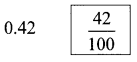(c)Solution: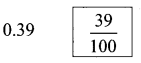(d)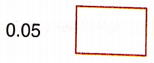Solution:(e)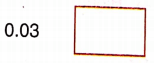Solution:(f)Solution: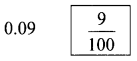(g)Solution: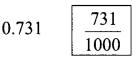(h)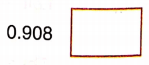Solution:(i)Solution:DAV Class 5 Maths Chapter 5 Worksheet 3 Notes

Convert Fractions to Decimals
Convert $$\frac{87}{1000}$$ into decimals.
Step 1. See denominator.Step 2. In the numerator, count three digits from the right and move towards the left, then, put a point (.).Since there are only two digits, we will put a zero and then, a decimal.